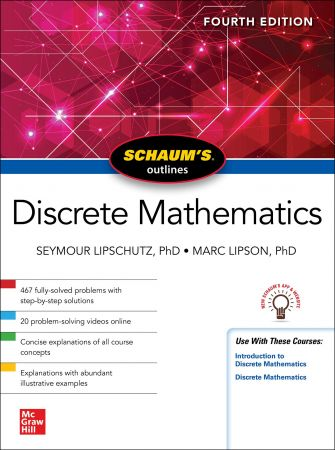# Schaum’s Outline of Discrete Mathematics, 4th EditionEnglish | November 30th, 2021 | ISBN: 1264258801 | 496 pages | True EPUB | 52.06 MB

Study smarter and stay on top of your discrete mathematics course with the bestselling Schaum’s Outline-now with the NEW Schaum’s app and website!

Schaum’s Outline of Discrete Mathematics, Fourth Edition is the go-to study guide for more than 115,000 math majors and first- and second-year university students taking basic computer science courses. With an outline format that facilitates quick and easy review, Schaum’s Outline of Discrete Mathematics, Fourth Edition helps you understand basic concepts and get the extra practice you need to excel in these courses.

Coverage includes set theory; relations; functions and algorithms; logic and propositional calculus; techniques of counting; advanced counting techniques, recursion; probability; graph theory; directed graphs; binary trees; properties of the integers; languages, automata, machines; finite state machines and Turning machines; ordered sets and lattices, and Boolean algebra.

Features
• NEW to this edition: the new Schaum’s app and website!
• NEW to this edition: 20 NEW problem-solving videos online
• 467 solved problems, and hundreds of additional practice problems
• Outline format to provide a concise guide to the standard college course in discrete mathematics
• Clear, concise explanations of discrete mathematics concepts
• Expanded coverage of logic, the rules of inference and basic types of proofs in mathematical reasoning
• Increased emphasis on discrete probability and aspects of probability theory, and greater accessibility to counting techniques.
• Logic chapter emphasizes the IF-THEN and IF-THEN-ELSE sequencing that occurs in computer programming
• Computer arithmetic chapter covers binary and hexagon addition and multiplication
• Cryptology chapter includes substitution and RSA method
• Supports these major texts: Discrete Mathematics and Its Applications (Rosen), and Discrete Mathematics (Epp)
• Appropriate for the following courses: Introductory Discrete Mathematics and Discrete Mathematics